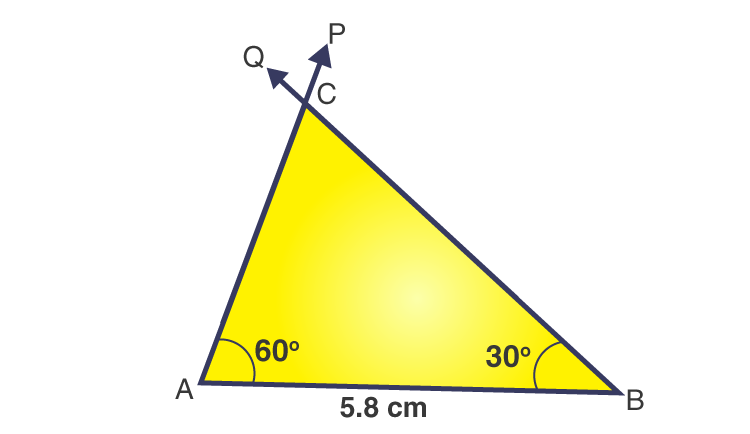# Construct ΔABC, given m ∠A =60o, m ∠B = 30o and AB = 5.8 cm.

SolutionThe steps for construction are given below:

1. Draw a line segment AB = 5.8 cm.

2. From point A, draw a ray P to making an angle of 60o i.e. ∠PAB = 60o.

3. From point B, draw a ray Q to making an angle of 30o i.e. ∠QBA = 30o.

4. Now the two rays AP and BQ intersect From the point C.

Then, ΔABC obtained is the required triangle.(0)(0)